### Baldur's GateHover over the thumbnail for a full-size version.

Author ghazbaran author:ghazbaran n-art nonplayable rated 2007-11-26 4 by 11 people. \$Baldur's Gate#ghazbaran#none#KDC<;LKDC<;LKDC<;LKDCLKMJEB=:MJEB=:MJEB=:MJEBMLKDC<;LKDC<;LKDC<;LKDCL:MJEB=:MJEB=:MJEB=:MJEB;LKDC<;LKDC<;LKDC<;LKDC=:MJEB=:MJEB=:MJEB=:MJE<;LKDC<;LKDC<;LKDC<;LKD1MQQQQQQQQQQQQQQQQQQQJ1E000000000000000000000BP000000000000000000000NP000000000000000000000NP000000000000000000000NP000000000000000000000NP000000000000000000000NP000000000000000000000NP000000000000000000000NP000000000000000000000NP000000000000000000000NP000000000000000000000NP000000000000000000000NP000000000000000000000NP000000000000000000000ND000000000000000000000C1LOOOOOOOOOOOOOOOOOOOK1=:MJEB=:MJEB=:MJEB=:MJE<;LKDC<;LKDC<;LKDC<;LKD:MJEB=:MJEB=:MJEB=:MJEB;LKDC<;LKDC<;LKDC<;LKDCMJEB=:MJEB=:MJEB=:MJEBMLKDC<;LKDC<;LKDC<;LKDCLJEB=:MJEB=:MJEB=:MJEB=:|10^396,312!10^390,312!10^384,312!10^378,312!10^372,312!10^372,324!10^372,318!10^372,330!10^372,336!10^372,342!10^372,306!10^378,306!10^384,306!10^390,306!10^396,306!10^396,300!10^390,300!10^378,300!10^372,300!10^390,300!10^384,300!10^378,342!10^378,348!10^378,354!10^378,360!10^372,366!10^372,372!10^366,378!10^360,384!10^354,390!10^354,396!10^348,402!10^348,408!10^348,414!10^348,420!10^348,426!10^354,432!10^354,438!10^360,444!10^354,456!10^360,456!10^354,462!10^354,468!10^354,474!10^348,480!10^348,486!10^348,492!10^342,498!10^342,504!10^342,510!10^342,516!10^336,522!10^336,528!10^336,534!10^336,540!10^330,546!10^330,552!10^330,558!10^330,564!10^336,564!10^342,564!10^336,546!10^336,552!10^336,558!10^342,552!10^342,546!3^348,546!3^348,552!3^348,558!3^348,564!3^354,564!3^354,558!3^354,552!3^354,546!10^342,540!10^342,534!10^342,534!10^342,528!10^348,540!10^348,534!10^348,534!10^348,528!10^354,522!10^354,522!10^354,516!10^354,516!10^354,510!10^354,504!10^354,498!10^360,498!10^360,492!10^360,486!10^360,480!10^366,474!10^366,468!10^366,462!10^372,456!10^378,456!10^384,456!10^390,456!10^396,456!10^402,456!10^408,456!10^414,456!10^420,456!10^426,456!10^432,462!10^432,468!10^438,474!10^438,480!10^444,486!10^444,492!10^450,498!10^450,504!10^456,510!10^456,516!10^462,522!10^462,528!10^462,534!10^456,534!10^462,540!10^468,546!10^468,552!10^468,558!10^474,564!10^468,564!10^474,564!10^480,564!10^480,564!10^480,558!10^480,552!10^480,546!10^474,540!10^474,534!10^474,528!10^474,522!10^468,516!10^468,510!10^462,504!10^462,498!10^456,492!10^456,486!10^450,480!10^450,474!10^444,468!10^444,462!10^432,456!10^438,468!10^438,474!10^438,462!10^444,474!10^444,480!10^444,486!10^450,486!10^450,492!10^450,498!10^456,498!10^456,504!10^456,510!10^462,510!10^462,516!10^462,522!10^468,522!10^468,528!10^468,534!10^468,546!10^474,546!10^474,552!10^480,558!10^336,522!10^342,522!10^342,522!10^348,522!10^348,522!10^348,510!10^348,510!10^348,504!10^348,492!10^348,498!10^354,492!10^354,486!10^354,486!10^354,480!10^360,474!10^354,462!10^360,468!10^360,462!10^366,450!10^438,456!10^432,450!10^432,444!10^432,438!10^432,432!10^426,426!10^426,420!10^426,414!10^426,408!10^420,402!10^420,396!10^420,384!10^420,384!10^420,390!10^414,378!10^414,366!10^414,372!10^414,360!10^414,354!10^408,348!10^408,342!10^408,336!10^408,330!10^408,324!10^408,324!10^408,318!10^408,312!10^408,306!10^408,300!10^408,300!10^402,300!10^402,306!10^402,312!10^414,324!10^414,318!10^414,312!10^414,306!10^414,300!10^420,300!10^426,294!10^426,300!10^426,288!10^420,282!10^414,276!10^414,270!10^408,264!10^408,258!10^372,294!10^372,294!10^372,288!10^372,282!10^372,276!10^372,264!10^372,276!10^372,270!10^372,258!10^378,258!10^384,258!10^384,258!10^396,258!10^396,258!10^390,258!10^390,264!10^384,264!10^384,264!10^384,264!10^384,270!10^384,276!10^384,276!10^384,276!10^384,282!10^384,282!10^384,282!10^390,282!10^396,282!10^402,282!10^414,282!10^414,288!10^426,294!10^414,294!10^414,294!10^414,294!10^408,294!10^402,294!10^402,294!10^396,294!10^396,294!10^390,294!10^384,294!10^378,294!10^384,294!10^378,294!10^378,288!10^378,288!10^378,282!10^378,282!10^378,270!10^378,270!10^378,276!10^378,276!10^384,288!10^390,288!10^396,288!10^396,288!10^402,288!10^408,288!10^408,276!10^408,276!10^408,270!10^402,270!10^396,270!10^390,270!10^390,270!10^390,276!10^396,276!10^402,276!10^402,258!10^420,294!10^396,264!10^408,264!10^402,264!10^402,264!10^390,264!10^378,264!10^378,264!10^408,288!10^408,282!10^420,282!10^420,288!10^348,516!10^474,540!10^474,540!10^468,540!10^480,552!10^480,558!10^480,558!10^474,552!10^474,558!10^474,558!10^474,540!10^468,528!10^462,516!10^456,504!10^444,492!10^378,318!10^378,318!10^378,324!10^378,330!10^378,336!10^378,336!10^390,330!10^390,336!10^390,336!10^384,336!10^384,336!10^390,336!10^390,330!10^384,330!10^384,318!10^384,324!10^396,324!10^396,324!10^390,324!10^390,318!10^396,318!10^402,318!10^402,318!10^402,324!10^402,330!10^396,336!10^396,342!10^402,342!10^402,342!10^396,342!10^396,342!10^396,342!10^390,342!10^390,342!10^384,348!10^384,342!10^384,348!10^390,348!10^390,354!10^390,354!10^396,354!10^396,354!10^402,354!10^402,354!10^402,354!10^402,354!10^402,348!10^396,348!10^396,348!10^384,354!10^384,354!10^384,360!10^384,360!10^384,366!10^384,366!10^390,366!10^390,366!10^396,366!10^396,366!10^402,366!10^408,366!10^408,354!10^408,354!10^408,360!10^402,360!10^402,360!10^396,360!10^396,360!10^390,360!10^396,330!10^402,336!10^390,324!10^384,324!10^384,324!10^396,330!10^396,318!10^396,312!10^396,312!10^390,312!10^390,312!10^384,312!10^378,312!10^378,312!10^372,312!10^390,318!10^384,318!10^378,318!10^372,318!10^396,336!10^390,336!10^384,336!10^474,534!10^462,534!10^462,528!3^486,564!3^486,558!3^486,552!3^492,564!3^492,558!3^492,552!3^492,546!3^486,540!3^486,534!3^486,528!3^486,522!3^480,516!3^480,510!3^474,504!3^474,498!3^468,492!3^468,486!3^462,480!3^462,474!3^456,468!3^450,462!3^444,456!3^360,540!3^360,534!3^360,528!3^366,522!3^366,516!3^366,510!3^366,504!3^372,498!3^372,492!3^372,486!3^372,486!3^372,480!3^378,474!3^378,474!3^378,474!3^378,468!3^372,468!3^372,474!3^372,480!3^366,492!3^366,498!3^360,504!3^360,510!3^360,510!3^360,516!3^360,516!3^360,522!3^360,528!3^360,528!3^360,534!3^360,534!3^360,534!3^354,534!3^354,534!3^354,540!3^354,546!3^354,552!3^348,558!3^348,564!3^348,558!3^348,558!3^348,552!3^354,540!3^360,510!3^366,492!3^366,492!3^372,486!3^372,486!3^366,516!3^360,534!3^360,534!3^360,528!3^354,528!3^354,534!3^372,486!3^372,486!3^372,486!3^366,486!3^366,486!3^372,480!3^378,468!3^378,462!3^372,462!3^450,462!3^450,468!3^450,468!3^450,474!3^456,474!3^456,474!3^462,480!3^462,480!3^462,486!3^462,492!3^468,492!3^468,498!3^468,498!3^474,504!3^474,510!3^474,510!3^474,516!3^480,516!3^480,522!3^474,522!3^474,534!3^480,534!3^486,546!3^486,546!3^486,552!3^474,540!3^444,450!3^444,444!3^444,444!3^444,438!3^444,438!3^444,432!3^438,426!3^438,420!3^438,414!3^438,408!3^432,402!3^432,396!3^432,384!3^432,390!3^426,378!3^426,372!3^426,366!3^426,360!3^426,354!3^420,348!3^420,342!3^420,336!3^426,330!3^426,324!3^426,324!3^426,318!3^426,312!3^432,306!3^438,300!3^438,294!3^438,288!3^432,282!3^426,282!3^420,270!3^426,270!3^426,270!3^426,276!3^432,276!3^438,282!3^438,288!3^432,288!3^432,294!3^432,294!3^432,300!3^432,300!3^432,306!3^432,306!3^426,312!3^426,312!3^426,312!3^426,312!3^420,312!3^426,306!3^426,306!3^420,312!3^420,324!3^420,324!3^420,324!3^420,330!3^420,336!3^414,336!3^414,342!3^414,348!3^414,354!3^414,360!3^420,360!3^420,366!3^426,360!3^426,372!3^426,378!3^420,384!3^432,396!3^432,396!3^432,402!3^432,408!3^438,414!3^438,420!3^438,432!3^438,444!3^444,438!3^444,438!3^438,432!3^432,414!3^432,402!3^432,390!3^432,384!3^426,372!3^426,366!3^420,354!3^414,342!3^414,330!3^414,324!3^420,312!3^420,306!3^486,528!3^438,444!3^438,438!3^438,438!3^438,432!3^432,414!3^426,402!3^420,396!3^420,390!3^426,390!3^426,396!3^420,378!3^420,372!3^420,366!3^480,534!10^480,534!3^420,264!3^420,258!3^414,258!3^414,270!3^414,252!3^408,246!3^402,240!10^372,252!10^378,246!10^378,252!10^390,252!10^390,252!10^396,252!10^396,252!3^402,252!3^402,246!3^396,246!3^408,252!10^384,246!10^384,246!10^390,246!10^396,240!10^396,240!10^390,240!10^378,372!10^384,366!10^378,372!10^378,372!10^378,366!10^378,378!10^372,378!10^372,378!10^372,384!10^372,384!10^372,390!10^366,390!10^366,390!10^366,402!10^384,408!10^384,408!10^384,420!10^384,420!10^372,432!10^372,432!10^360,420!10^360,408!10^360,408!10^384,420!10^384,420!10^360,420!10^384,420!10^384,408!10^372,408!10^372,420!10^372,408!10^372,396!10^384,396!10^384,396!10^384,396!10^384,384!10^396,384!10^396,384!10^396,372!10^384,372!10^396,372!10^396,372!10^408,384!10^408,384!10^408,372!10^408,384!10^408,396!10^408,408!10^408,420!10^408,432!10^420,432!10^420,432!10^420,444!10^420,444!10^408,444!10^408,432!10^420,420!10^420,396!10^420,420!10^420,408!10^408,444!10^408,444!10^396,444!10^396,444!10^384,444!10^384,444!10^384,444!10^372,444!10^360,432!10^372,432!10^384,432!10^384,432!10^396,432!10^396,420!10^396,408!10^396,408!10^396,396!10^402,378!10^402,378!10^396,378!10^390,378!10^384,384!10^390,390!10^390,396!10^384,402!10^384,402!10^378,408!10^378,414!10^378,420!10^372,426!10^366,432!10^366,432!10^378,438!10^378,438!10^378,450!10^378,456!10^372,444!10^366,444!10^366,432!10^366,426!10^360,420!10^360,420!10^360,414!10^366,414!10^360,408!10^360,396!10^372,396!10^384,384!10^384,384!10^378,396!10^372,402!10^372,408!10^378,396!10^384,372!10^384,372!10^384,384!10^384,384!10^396,384!10^402,384!10^414,384!10^414,384!10^402,384!10^402,384!10^390,390!10^378,396!10^366,402!10^360,408!10^354,414!10^354,420!10^354,420!10^354,426!10^354,426!10^366,432!10^366,432!10^366,432!10^366,414!10^372,414!10^372,408!10^372,408!10^360,402!10^360,402!10^354,402!10^360,408!10^384,402!10^384,396!10^378,384!10^378,384!10^384,390!10^384,378!10^384,384!10^384,384!10^402,378!10^402,378!10^402,372!10^390,372!10^390,384!10^390,384!10^378,390!10^378,390!10^372,396!10^366,408!10^366,408!10^366,414!10^366,420!10^366,426!10^366,420!10^366,420!10^360,426!10^360,438!10^360,438!10^372,438!10^372,438!10^384,450!10^378,450!10^372,444!10^366,432!10^360,432!10^366,420!10^366,414!10^372,402!10^378,396!10^378,402!10^354,414!10^354,402!10^354,408!10^366,396!10^366,384!10^366,396!10^366,438!10^360,438!10^366,438!10^366,444!10^372,450!10^378,444!10^378,444!10^384,444!10^384,444!10^390,444!10^390,444!10^390,450!10^390,450!10^396,450!10^396,450!10^402,450!10^408,450!10^402,450!10^414,444!10^414,444!10^414,450!10^420,450!10^420,450!10^426,450!10^426,450!10^426,444!10^426,444!10^426,438!10^420,438!10^420,438!10^414,438!10^414,438!10^408,438!10^408,438!10^408,438!10^402,438!10^402,438!10^402,438!10^396,438!10^390,438!10^390,438!10^390,438!10^390,432!10^390,426!10^384,426!10^378,426!10^384,426!10^390,426!10^396,426!10^396,426!10^402,426!10^390,438!10^384,438!10^378,438!10^390,432!10^396,432!10^408,432!10^408,432!10^414,432!10^420,432!10^426,432!10^414,420!10^414,420!10^408,426!10^408,426!10^402,426!10^402,432!10^402,444!10^378,432!10^396,414!10^408,414!10^366,480!10^408,420!10^402,408!10^390,414!10^390,414!10^390,408!10^390,402!10^384,408!10^384,408!10^390,414!10^396,414!10^402,414!10^396,420!10^378,420!10^384,414!10^384,414!10^360,390!10^420,426!10^402,420!10^390,420!10^414,426!10^414,420!10^420,414!10^420,408!10^414,408!10^414,408!10^414,408!10^414,414!10^414,414!10^420,408!10^420,402!10^420,402!10^420,402!10^414,396!10^414,390!10^408,390!10^408,390!10^408,396!10^408,402!10^408,402!10^408,408!10^402,408!10^402,408!10^402,408!10^402,396!10^402,390!10^402,390!10^402,402!10^390,402!10^390,396!10^396,396!10^396,390!10^396,402!10^414,402!10^408,378!10^360,456!10^360,450!10^360,450!10^360,444!10^372,456!10^366,456!3^426,348!3^426,330!3^426,330!3^426,342!3^426,342!10^384,240!10^366,246!10^360,240!10^354,234!10^348,228!10^342,222!10^336,216!10^330,210!10^330,204!10^336,198!10^330,198!10^336,192!10^336,186!10^336,186!10^336,180!10^336,174!10^336,168!10^336,210!10^336,204!10^336,192!10^336,198!10^330,198!10^330,192!10^330,186!10^330,186!10^330,174!10^330,168!10^330,168!10^336,156!10^336,156!10^336,162!10^336,156!10^336,150!10^336,144!10^336,144!10^336,144!10^330,132!10^330,132!10^330,138!10^330,144!10^330,126!10^330,126!10^330,126!10^324,126!10^324,120!10^324,114!10^336,120!10^336,114!10^336,108!10^330,108!10^324,108!10^330,120!10^330,120!10^336,114!10^324,114!10^330,114!10^330,150!10^330,168!2^348,132,0.707106781186547,0.707106781186547!2^348,132,-0.707106781186547,-0.707106781186547!2^348,132,-0.707106781186547,0.707106781186547!2^348,132,0.707106781186547,-0.707106781186547!2^342,126,0.707106781186547,-0.707106781186547!2^336,120,0.707106781186547,-0.707106781186547!2^330,114,0.707106781186547,-0.707106781186547!2^342,126,-0.707106781186547,0.707106781186547!2^336,120,-0.707106781186547,0.707106781186547!2^330,114,-0.707106781186547,0.707106781186547!2^318,114,-0.707106781186547,-0.707106781186547!2^312,108,0.707106781186547,0.707106781186547!2^318,102,0.707106781186547,0.707106781186547!2^324,96,0.707106781186547,0.707106781186547!2^324,108,-0.707106781186547,-0.707106781186547!2^330,102,-0.707106781186547,-0.707106781186547!2^318,114,-0.707106781186547,-0.707106781186547!2^312,120,-0.707106781186547,-0.707106781186547!2^336,96,-0.707106781186547,-0.707106781186547!2^330,90,0.707106781186547,0.707106781186547!2^324,96,0.707106781186547,0.707106781186547!2^318,102,0.707106781186547,0.707106781186547!2^312,108,0.707106781186547,0.707106781186547!2^306,114,0.707106781186547,0.707106781186547!2^324,96,0.707106781186547,-0.707106781186547!2^318,90,0.707106781186547,-0.707106781186547!2^312,84,0.707106781186547,-0.707106781186547!2^306,78,0.707106781186547,-0.707106781186547!2^300,72,0.707106781186547,-0.707106781186547!2^294,66,0.707106781186547,-0.707106781186547!2^288,60,0.707106781186547,-0.707106781186547!2^282,54,0.707106781186547,-0.707106781186547!2^276,48,0.707106781186547,-0.707106781186547!2^270,42,0.707106781186547,-0.707106781186547!2^312,108,-0.707106781186547,0.707106781186547!2^306,102,-0.707106781186547,0.707106781186547!2^300,96,-0.707106781186547,0.707106781186547!2^294,90,-0.707106781186547,0.707106781186547!2^288,84,-0.707106781186547,0.707106781186547!2^282,78,-0.707106781186547,0.707106781186547!2^276,72,-0.707106781186547,0.707106781186547!2^270,66,-0.707106781186547,0.707106781186547!2^264,60,-0.707106781186547,0.707106781186547!2^258,54,-0.707106781186547,0.707106781186547!2^252,48,-0.707106781186547,0.707106781186547!2^246,42,-0.707106781186547,0.707106781186547!2^240,36,-0.707106781186547,0.707106781186547!2^252,36,-0.707106781186547,0.707106781186547!2^246,36,-0.707106781186547,0.707106781186547!2^252,42,-0.707106781186547,0.707106781186547!2^258,48,-0.707106781186547,0.707106781186547!2^258,36,-0.707106781186547,0.707106781186547!2^264,42,-0.707106781186547,0.707106781186547!2^270,48,-0.707106781186547,0.707106781186547!2^276,54,-0.707106781186547,0.707106781186547!2^282,60,-0.707106781186547,0.707106781186547!2^288,66,-0.707106781186547,0.707106781186547!2^294,72,-0.707106781186547,0.707106781186547!2^300,78,-0.707106781186547,0.707106781186547!2^306,84,-0.707106781186547,0.707106781186547!2^312,90,-0.707106781186547,0.707106781186547!2^318,96,-0.707106781186547,0.707106781186547!2^312,102,-0.707106781186547,0.707106781186547!2^312,102,-0.707106781186547,0.707106781186547!2^312,96,-0.707106781186547,0.707106781186547!2^306,90,-0.707106781186547,0.707106781186547!2^306,90,-0.707106781186547,0.707106781186547!2^300,90,-0.707106781186547,0.707106781186547!2^300,84,-0.707106781186547,0.707106781186547!2^294,78,-0.707106781186547,0.707106781186547!2^294,78,-0.707106781186547,0.707106781186547!2^288,78,-0.707106781186547,0.707106781186547!2^288,66,-0.707106781186547,0.707106781186547!2^288,72,-0.707106781186547,0.707106781186547!2^282,72,-0.707106781186547,0.707106781186547!2^282,72,-0.707106781186547,0.707106781186547!2^282,66,-0.707106781186547,0.707106781186547!2^276,66,-0.707106781186547,0.707106781186547!2^276,60,-0.707106781186547,0.707106781186547!2^270,60,-0.707106781186547,0.707106781186547!2^264,54,-0.707106781186547,0.707106781186547!2^264,54,-0.707106781186547,0.707106781186547!2^264,48,-0.707106781186547,0.707106781186547!2^264,42,-0.707106781186547,0.707106781186547!2^282,54,-0.707106781186547,0.707106781186547!2^282,48,-0.707106781186547,0.707106781186547!2^276,48,-0.707106781186547,0.707106781186547!2^276,48,-0.707106781186547,0.707106781186547!2^270,42,-0.707106781186547,0.707106781186547!2^270,36,-0.707106781186547,0.707106781186547!2^264,36,-0.707106781186547,0.707106781186547!3^378,234!3^372,234!3^372,228!3^366,222!3^360,216!3^354,210!3^348,204!3^348,198!3^348,192!3^348,186!3^348,180!3^372,240!3^372,240!3^372,240!3^366,234!3^366,234!3^366,234!3^366,234!3^360,228!3^360,228!3^354,228!3^354,222!3^348,222!3^348,216!3^348,216!3^342,216!3^342,210!3^348,210!3^348,210!3^354,216!3^354,216!3^354,222!3^366,228!3^366,228!3^372,234!3^372,234!3^372,228!3^366,228!3^360,222!3^360,222!3^360,222!3^366,234!3^372,240!3^342,204!3^342,204!3^342,198!3^342,192!3^342,186!3^342,180!3^342,180!3^342,174!3^342,168!3^342,168!3^342,162!3^342,162!3^342,156!3^342,150!3^342,150!10^330,162!10^330,156!10^330,168!10^330,168!10^330,174!10^330,180!10^330,180!10^336,138!10^336,132!3^342,144!3^342,138!3^342,138!2^348,132,-0.707106781186547,-0.707106781186547!2^348,132,0.707106781186547,0.707106781186547!2^348,132,-0.707106781186547,0.707106781186547!2^348,132,0.707106781186547,-0.707106781186547!2^342,126,-0.707106781186547,0.707106781186547!2^336,120,-0.707106781186547,0.707106781186547!10^336,126!10^336,126!10^336,120!10^336,120!10^336,114!10^336,114!10^330,114!10^330,120!3^432,432!3^432,426!3^438,426!3^432,408!3^426,396!3^426,384!3^426,384!3^426,372!3^426,336!3^426,306!3^426,306!3^426,312!10^426,426!10^432,426!3^408,228!3^414,222!3^414,222!3^414,216!3^414,210!3^414,204!3^402,228!3^396,228!10^390,228!10^384,222!10^378,216!10^378,210!10^378,204!10^378,204!10^378,198!10^378,198!10^378,192!10^378,186!10^378,186!10^378,186!10^378,180!10^396,216!10^396,210!10^402,204!10^402,204!10^402,198!10^384,174!10^390,174!10^402,174!10^402,174!10^396,174!10^396,174!10^402,180!10^402,180!10^402,186!10^396,186!10^396,186!10^390,186!10^390,186!10^390,186!10^390,180!10^396,180!10^390,180!10^396,192!10^390,186!10^390,192!10^390,204!10^396,192!10^390,192!10^390,198!10^384,204!10^384,216!10^384,216!10^390,216!10^384,204!10^384,204!10^384,192!10^384,186!10^384,180!10^384,192!10^384,204!10^384,210!10^390,210!10^402,198!10^402,198!10^396,198!10^378,204!10^378,198!10^384,198!3^408,216!3^402,216!3^396,216!3^402,216!3^402,216!3^408,216!3^408,210!3^402,210!3^402,210!3^414,192!3^414,186!3^414,186!3^414,180!3^414,198!3^408,174!3^408,186!3^408,192!3^408,192!3^408,210!3^408,204!3^408,180!3^408,192!3^408,198!3^408,204!3^408,216!3^408,222!3^408,222!3^402,222!3^396,222!3^390,222!3^408,222!3^402,216!10^384,168!10^390,168!10^390,168!10^390,168!10^384,162!10^384,162!10^378,156!10^372,156!10^366,156!10^360,162!10^360,168!10^360,168!10^360,174!10^360,180!10^366,174!10^366,174!10^366,174!10^366,168!10^366,162!10^372,162!10^384,162!10^354,168!10^354,168!10^354,174!10^354,186!10^366,186!10^366,192!10^366,198!10^366,210!10^366,204!10^366,204!10^360,204!10^360,198!10^354,192!10^366,180!10^360,186!10^360,186!10^360,186!10^354,180!10^372,168!10^348,240!10^348,240!10^348,246!10^348,246!10^348,252!10^348,252!10^348,258!10^348,264!10^348,264!10^348,264!10^348,258!10^348,258!10^360,252!10^360,258!10^360,258!10^360,258!10^360,264!10^360,264!10^360,276!10^360,276!10^360,276!10^360,276!10^360,270!10^360,282!10^360,282!10^360,288!10^360,294!10^354,282!10^354,288!10^354,288!10^348,282!10^348,282!10^348,282!10^348,282!10^348,276!10^348,270!10^348,270!10^348,270!10^354,270!10^354,270!10^354,264!10^354,252!10^354,246!10^354,240!10^342,234!10^342,246!10^342,246!10^342,252!10^342,252!10^342,252!10^342,258!10^342,264!10^342,264!10^342,270!10^342,270!10^342,282!10^342,282!10^342,288!10^342,234!10^342,228!10^342,240!10^342,276!10^402,174!10^414,174!10^414,174!10^408,168!10^402,168!10^396,168!10^396,168!10^348,300!10^348,294!10^348,294!10^348,300!10^348,306!10^348,312!10^438,354!10^438,348!10^444,348!10^450,354!10^456,354!10^462,360!10^450,360!10^450,360!10^456,360!10^456,360!10^450,360!10^444,360!10^444,360!10^444,354!10^456,366!10^462,366!10^462,366!3^462,348!3^462,348!3^462,342!3^462,336!3^462,330!3^474,342!3^480,354!3^486,354!3^486,354!3^474,360!3^468,348!3^468,348!3^468,348!3^468,348!3^462,354!3^474,354!3^474,354!3^474,348!3^474,348!3^480,336!3^474,366!3^468,366!3^468,366!3^468,366!10^438,354!3^432,342!3^438,342!3^444,342!3^444,342!3^450,348!3^456,348!10^438,360!10^438,360!10^432,354!10^372,246!10^372,246!10^372,246!10^372,252!10^372,252!3^438,336!3^432,330!10^444,366!10^450,372!10^456,378!10^462,372!10^432,348!10^432,348!10^420,348!10^420,348!10^420,342!10^420,342!10^426,342!10^426,342!10^426,342!10^420,336!10^420,324!10^420,324!10^420,324!10^426,330!10^426,336!10^438,348!10^438,348!10^426,336!10^426,324!10^426,336!10^420,348!10^420,342!10^420,342!10^396,204!10^390,204!10^390,204!0^384,504!0^396,504!0^408,504!0^420,504!0^432,504!0^480,492!0^492,480!0^504,468!0^516,444!0^516,432!0^516,420!0^516,408!0^516,396!0^516,384!0^516,372!0^516,456!0^324,504!0^312,492!0^300,480!0^288,468!0^288,456!0^288,444!0^288,432!0^288,432!0^288,420!0^288,408!0^288,396!0^288,384!0^288,384!0^288,372!0^288,360!0^288,348!0^288,336!0^288,324!0^288,312!0^288,300!0^288,288!0^288,276!0^288,264!0^288,252!0^288,240!0^300,228!0^312,216!0^432,180!0^444,180!0^516,360!0^516,348!0^516,348!0^516,336!0^516,324!0^516,312!0^516,312!0^516,300!0^516,300!0^516,288!0^516,276!0^516,276!0^516,276!0^516,264!0^516,252!0^516,240!0^504,228!0^492,216!0^480,204!0^468,192!0^444,180!0^456,180!0^492,204!0^504,192!0^516,180!0^480,192!0^492,192!0^504,180!0^492,180!0^480,180!0^468,180!0^504,168!0^516,168!0^528,156!0^540,144!0^528,180!0^540,168!0^552,156!0^504,156!0^516,144!0^516,132!0^480,168!0^480,156!0^480,144!0^480,132!0^456,168!0^456,156!0^456,144!0^456,144!0^456,132!0^432,132!0^432,144!0^432,144!0^432,156!0^432,156!0^432,168!0^408,132!0^408,144!0^384,132!0^384,144!0^408,156!0^360,132!0^360,144!0^300,168!0^300,180!0^312,192!0^312,192!0^312,204!0^276,180!0^276,192!0^288,192!0^288,204!0^300,216!0^276,228!0^276,228!0^276,228!0^288,240!0^264,228!0^252,216!0^252,252!0^264,252!0^276,264!0^276,264!0^276,312!0^276,312!0^240,252!0^276,288!0^264,288!0^252,288!0^252,288!0^240,288!0^240,288!0^264,312!0^252,312!0^240,324!0^276,348!0^264,348!0^252,348!0^252,348!0^240,348!0^276,384!0^264,384!0^252,384!0^252,384!0^252,384!0^276,408!0^264,408!0^252,408!0^252,408!0^276,432!0^264,432!0^264,432!0^252,432!0^276,456!0^264,456!0^264,456!0^252,456!0^276,480!0^276,480!0^264,492!0^264,492!0^300,504!0^300,504!0^288,504!0^288,516!0^276,528!0^324,528!0^324,516!0^384,516!0^384,528!0^384,528!0^384,540!0^420,516!0^420,528!0^420,528!0^420,540!0^444,516!0^444,528!0^492,492!0^504,504!0^504,504!0^516,516!0^516,516!0^516,480!0^528,480!0^528,480!0^528,492!0^540,492!0^528,456!0^552,456!0^552,456!0^552,456!0^540,456!0^564,456!0^528,432!0^552,432!0^552,432!0^552,432!0^540,432!0^564,420!0^528,408!0^528,408!0^552,408!0^552,408!0^552,408!0^540,408!0^528,372!0^540,372!0^540,372!0^552,372!0^552,372!0^564,372!0^528,348!0^540,348!0^540,348!0^552,348!0^552,348!0^516,312!0^540,312!0^540,312!0^540,312!0^540,312!0^528,312!0^552,312!0^552,312!0^528,288!0^540,288!0^540,288!0^540,288!0^552,288!0^552,288!0^564,288!0^528,264!0^540,252!0^540,252!0^552,252!0^552,252!0^552,252!0^528,228!0^516,228!0^540,216!0^540,216!0^552,216!0^564,204!0^564,204!0^504,216!0^516,204!0^528,204!0^540,192!0^312,156!0^312,156!0^288,156!0^288,156!0^288,156!0^276,180!0^252,192!0^252,228!0^552,468!0^552,432!0^564,408!0^564,372!0^552,324!0^552,276!0^264,300!0^264,348!0^252,420!12^264,468!12^264,444!12^264,420!12^228,396!12^252,372!12^264,336!12^252,324!12^240,288!12^264,264!12^264,216!12^264,192!12^264,168!12^384,132!12^408,132!12^432,132!12^456,156!12^492,156!12^516,156!12^552,204!12^552,216!12^540,252!12^540,276!12^552,300!12^552,348!12^552,396!12^552,432!12^552,492!12^528,516!12^504,504!12^516,480!12^516,492!12^540,480!12^540,420!12^528,372!12^540,336!12^540,252!12^444,528!12^420,528!12^396,516!12^372,528!12^300,528!12^288,504!12^264,504!12^264,492!12^252,468!12^240,432!12^240,408!12^252,348!12^252,300!12^228,180!12^252,144!12^288,132!12^300,144!12^276,168!12^252,180!12^240,228!12^228,276!12^228,324!12^228,384!12^240,432!12^276,492!12^288,516!12^288,516!12^312,516!12^300,540!12^276,540!12^240,504!12^372,552!12^408,540!12^408,516!12^396,516!12^420,528!12^444,540!12^504,528!12^540,528!12^552,504!12^552,468!12^564,444!12^564,408!12^528,408!12^528,372!12^540,336!12^540,288!12^552,252!12^552,216!12^540,180!12^540,204!12^528,204!12^528,168!12^528,132!12^492,120!12^444,120!12^408,120!12^372,120!12^372,108!12^360,96!12^456,96!12^492,108!12^516,120!12^528,132!12^528,144!0^564,192!0^564,180!0^552,156!0^552,132!0^492,120!0^468,120!0^444,108!0^444,96!0^372,84!0^372,84!0^408,96!0^396,96!0^276,120!0^276,132!0^252,132!0^240,168!0^240,192!0^228,204!0^228,228!0^228,264!0^228,300!0^216,336!0^216,360!0^228,408!0^228,456!0^240,504!0^276,516!0^288,516!0^300,528!0^276,540!0^264,528!0^252,480!0^396,564!0^408,528!0^408,528!0^432,540!0^456,540!0^420,528!0^408,528!0^516,516!0^528,516!0^540,480!0^540,456!0^540,420!0^540,408!0^540,384!0^540,348!0^540,312!0^540,276!0^540,228!0^540,156!0^492,120!0^456,96!0^408,60!0^384,60!0^360,72!0^336,72!0^276,108!0^264,120!0^252,132!0^228,144!0^228,168!0^216,228!0^228,300!0^228,396!0^240,456!0^240,480!0^264,516!12^300,552!12^288,552!12^276,552!12^252,552!12^216,540!12^204,504!12^204,480!12^228,492!12^228,528!12^240,528!12^204,504!12^204,468!12^216,456!12^216,432!12^216,408!12^216,360!12^216,324!12^216,276!12^216,228!12^216,180!12^216,144!12^216,96!12^216,48!12^240,72!12^240,84!12^228,108!12^228,72!0^264,84!0^264,84!0^252,96!0^240,108!12^264,108!12^240,60!12^288,36!12^300,48!12^312,48!12^312,48!12^324,60!12^336,48!12^348,36!12^384,36!12^432,36!12^468,36!12^528,36!12^564,60!12^576,96!12^588,156!12^588,204!12^564,216!12^576,192!12^576,180!12^588,192!12^588,228!12^576,252!12^576,288!12^576,324!12^576,372!12^588,396!12^588,420!12^576,384!12^588,324!12^564,468!12^564,480!12^588,456!12^588,492!12^588,516!12^576,540!12^588,492!12^564,540!12^552,552!12^516,564!12^516,528!12^564,528!12^576,516!12^456,552!12^444,564!12^420,564!12^384,564!12^384,564!12^408,552!12^444,552!12^588,276!12^576,276!12^576,228!12^576,192!12^564,132!12^540,96!12^540,60!12^492,48!12^456,48!12^432,60!12^396,72!12^372,48!12^372,60!12^408,72!12^468,60!12^504,60!12^540,96!12^564,84!0^552,108!0^528,108!0^528,72!0^516,72!0^516,96!0^528,96!0^504,96!0^504,96!0^492,84!0^528,324!12^564,336!12^564,348!12^588,360!12^588,372!12^576,432!12^552,480!12^540,540!12^528,552!12^516,552!12^396,552!12^384,564!12^300,552!12^276,552!12^240,528!12^216,480!12^204,432!12^228,384!12^240,360!12^228,348!12^204,300!12^216,264!12^216,228!12^228,180!12^228,156!12^228,96!12^384,60!12^408,60!12^456,72!12^492,72!12^528,60!0^564,156!0^576,144!0^576,108!0^564,84!0^528,72!0^492,84!0^468,96!0^468,108!0^468,84!0^444,96!0^408,108!0^420,84!0^384,84!0^372,108!0^252,108!0^240,132!0^228,168!0^252,216!0^216,288!0^216,348!0^216,408!0^240,480!0^240,516!0^228,528!0^516,528!0^540,516!0^552,492!0^576,480!0^576,456!0^552,432!0^552,444!0^564,384!0^588,360!0^564,324!0^564,360!0^564,312!0^552,192!0^516,144!0^480,120!0^456,132!0^444,132!0^468,156!0^480,144!0^420,120!0^372,96!0^408,96!0^408,108!0^432,96!0^456,120!0^432,120!0^408,120!0^396,120!0^384,108!0^288,108!0^300,444!0^312,456!0^324,468!0^336,480!0^324,492!0^324,480!0^312,480!0^312,480!0^300,468!0^300,468!0^504,444!0^492,456!0^480,468!0^480,480!0^492,468!0^492,468!0^492,456!0^516,456!0^504,456!0^504,252!0^492,240!0^480,228!0^468,216!0^456,204!0^444,192!0^468,192!0^468,204!0^492,228!0^492,228!0^504,240!0^504,240!0^300,252!0^312,240!0^324,228!0^324,228!0^312,228!0^300,240# http://www.juegomania.org/Baldurs+Gate:+Dark+Alliance/fotos/gbadvance/0/30_c/Caratula+Baldurs+Gate:+Dark+Alliance.jpg

## Other maps by this author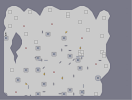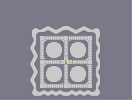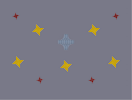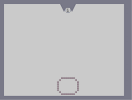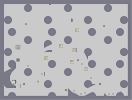Cave of a thousand wishes Outlaw Heaven 3D Rotating Triangle Sansui The Grim

Pages: (0)

### YEAH, no

I can't do that... I click till i bleed...

### With programs its easy to make arts.

Since the fun of making them is clicking until you bleed.
But the programs now, make it really easy...
I know many are not auto generated, but with programs you can copy and paste parts, fill a big part of the map with something and other things that make narting worse for the non program users (like me).

### i never really said about autogenerated

i was under the impression that you used a program to make your narts - i think it was one of your own descriptions that said it - so i must have misinterperated the actual use of the program.

### @ Cossack

why the hell does everyone think i use an art generator...
every friggin map i have made was placed object by object by me...
i dont believe in cheating... My program is a custom ned which i made to hold more objects so it doesn't lag...

i'm not offended but i just want people to know that i don't autogenerate my maps...

### altho

saying that - i dont mean NO3 is bad - he has good nart as well (just thought to clear that up - it wasnt a stab at NO3 in anyway :P)

### xept most of NO3's are generated by a program

this is reasonable but a little simple coz of the csnap - i just generally see csnap as a slightly inferior mode of outlining (but thats me :P) 3.5/5

### NO3 has done much better, Coenraad.

The figure looks nice in the thumbnail, 2.5/5

### Sorry

1.) For the dubble post (I hit back/forwards)
2.) I just went through ]{NO3's maps and I have to take back what I said below.

### Hey

Hey ']{NO3' I'd like to see you do better.

### Hey

Hey ']{NO3' I'd like to see you do better.

### fairly crap

i'll be generous and give you a 2.5

Thats fast.

5

### Ya I know the background sucks

but I have no time, did it in like 15 min